#Homogeneous Linear Equations With Constant CoefficientsConsider the nth-order linear equation with constant coefficients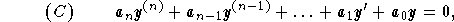with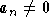. In order to generate n linearly independent solutions, we need to perform the following:

(1)
Write the characteristic equation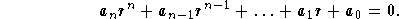Then, look for the roots. These roots will be of two natures: simple or multiple. Let us show how they generate independent solutions of the equation(H).

(2)
First case: Simple root
Let r be a simple root of the characteristic equation.
(2.1)
If r is a real number, then it generates the solution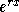;
(2.2)
If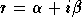is a complex root, then since the coefficients of the characteristic equation are real,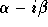is also a root. The two roots generate the two solutions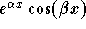and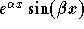;

(3)
Second case: Multiple root
Let r be a root of the characteristic equation with multiplicity m. If r is a real number, then generate the m independent solutions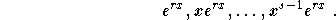Ifis a complex number, thenis also a root with multiplicity m. The two complex roots will generate 2m independent solutions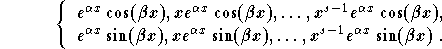Using properties of roots of polynomial equations, we will generate n independent solutions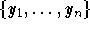. Hence, the general solution of the equation (H) is given by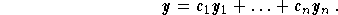Therefore, the real problem in solving (H) has to do more with finding roots of polynomial equations. We urge students to practice on this.

Example: Find the general solution of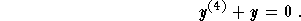Solution: Let us follow these steps:

(1)
Characteristic equation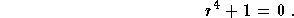Its roots are the complex numbers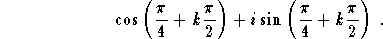In the analytical form, these roots are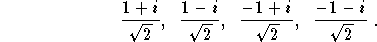;

(2)
Independent set of solutions
(2.1)
The complex roots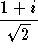and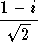generate the two solutions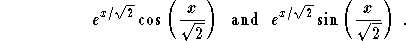;

(2.2)
The complex roots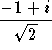and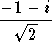generate the two solutions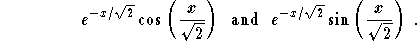;

(3)
The general solution is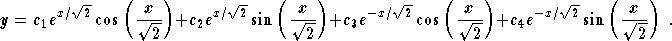As you may have noticed in this example, complex numbers do get involved very much in this kind of problem...[Differential Equations] [First Order D.E.] [Second Order D.E.]
[Geometry] [Algebra] [Trigonometry ]
[Calculus] [Complex Variables] [Matrix Algebra]S.O.S MATHematics home page

Do you need more help? Please post your question on our S.O.S. Mathematics CyberBoard.Author: Mohamed Amine Khamsi